## April 27, 2018

### Props in Network Theory

#### Posted by John Baez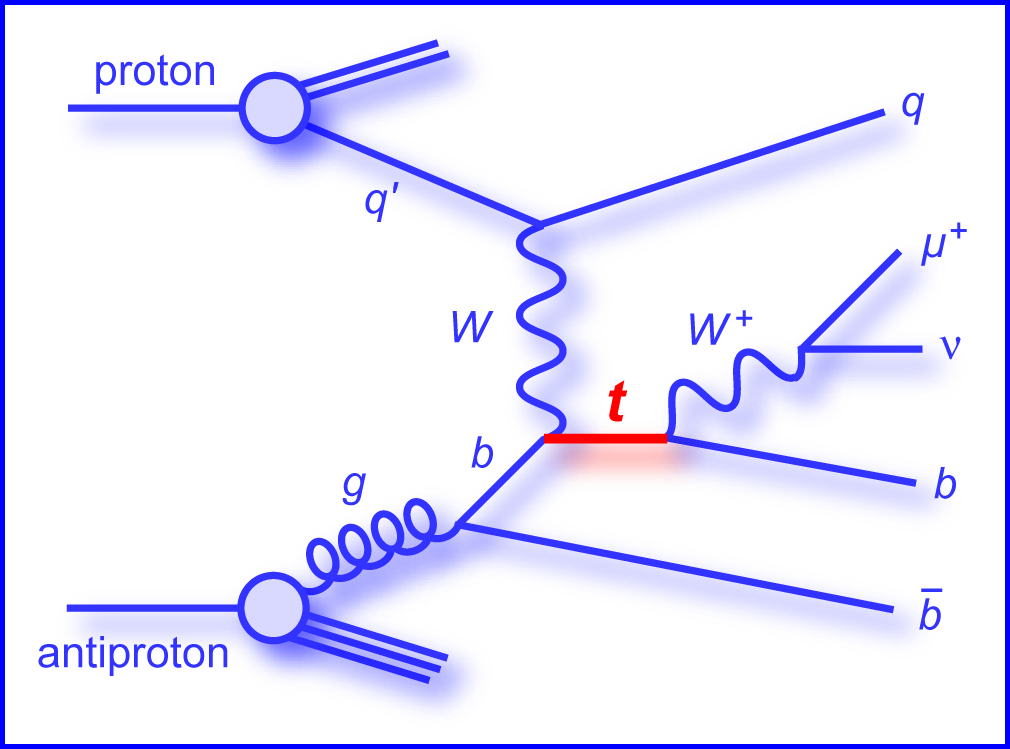Long before the invention of Feynman diagrams, engineers were using similar diagrams to reason about electrical circuits and more general networks containing mechanical, hydraulic, thermodynamic and chemical components. We can formalize this reasoning using ‘props’: that is, strict symmetric monoidal categories where the objects are natural numbers, with the tensor product of objects given by addition. In this approach, each kind of network corresponds to a prop, and each network of this kind is a morphism in that prop. A network with $m$ inputs and $n$ outputs is a morphism from $m$ to $n.$ Putting networks together in series is composition, and setting them side by side is tensoring.

In this paper, we study the props for various kinds of electrical circuits:

We start with circuits made solely of ideal perfectly conductive wires. Then we consider circuits with passive linear components like resistors, capacitors and inductors. Finally we turn on the power and consider circuits that also have voltage and current sources.

And here’s the cool part: each kind of circuit corresponds to a prop that pure mathematicians would eventually invent on their own! So, what’s good for engineers is often mathematically natural too.

We describe the ‘behavior’ of these various kinds of circuits using morphisms between props. In particular, we give a new proof of the black-boxing theorem proved earlier with Brendan Fong. Unlike the original proof, this new one easily generalizes to circuits with nonlinear components. We also use a morphism of props to clarify the relation between circuit diagrams and the signal-flow diagrams in control theory.

Here’s a quick sketch of the main ideas.

In his 1963 thesis, Lawvere introduced functorial semantics: the use of categories with specified extra structure as ‘theories’ whose ‘models’ are structure-preserving functors into other such categories:

In particular, a Lawvere theory is a category with finite cartesian products and a distinguished object $X$ such that every object is a power $X^n.$ These can serve as theories of mathematical structures that are sets $X$ equipped with $n$-ary operations

$f \colon X^n \to X$

obeying equational laws. However, structures of a more linear-algebraic nature are often vector spaces equipped with operations of the form

$f \colon X^{\otimes m} \to X^{\otimes n}$

To extend functorial semantics to these, Mac Lane introduced props—or as he called them, ‘PROPs’. The acronym stands for ‘products and permutations’:

• Saunders Mac Lane, Categorical algebra, Bulletin of the American Mathematical Society 71 (1965), 40–106.

A prop is a symmetric monoidal category equipped with a distinguished object $X$ such that every object is a tensor power $X^{\otimes n}.$ Working with tensor products rather than cartesian products puts operations having multiple outputs on an equal footing with operations having multiple inputs.

Already in 1949 Feynman had introduced his famous diagrams, which he used to describe theories of elementary particles. For a theory with just one type of particle, Feynman’s method amounts to specifying a prop where an operation $f \colon X^{\otimes m} \to X^{\otimes n}$ describes a process with $m$ particles coming in and $n$ going out. Although Feynman diagrams quickly caught on in physics, only in the 1980s did it become clear that they were a method of depicting morphisms in symmetric monoidal categories. A key step was the work of Joyal and Street, which rigorously justified reasoning in any symmetric monoidal category using ‘string diagrams’—a generalization of Feynman diagrams:

By now, many mathematical physicists are aware of props and the general idea of functorial semantics. In constrast, props seem to be virtually unknown in engineering!

But engineers have been using diagrammatic methods ever since the rise of electrical circuits. And in the 1940s, Olson explained how to apply circuit diagrams to networks of mechanical, hydraulic, thermodynamic and chemical components: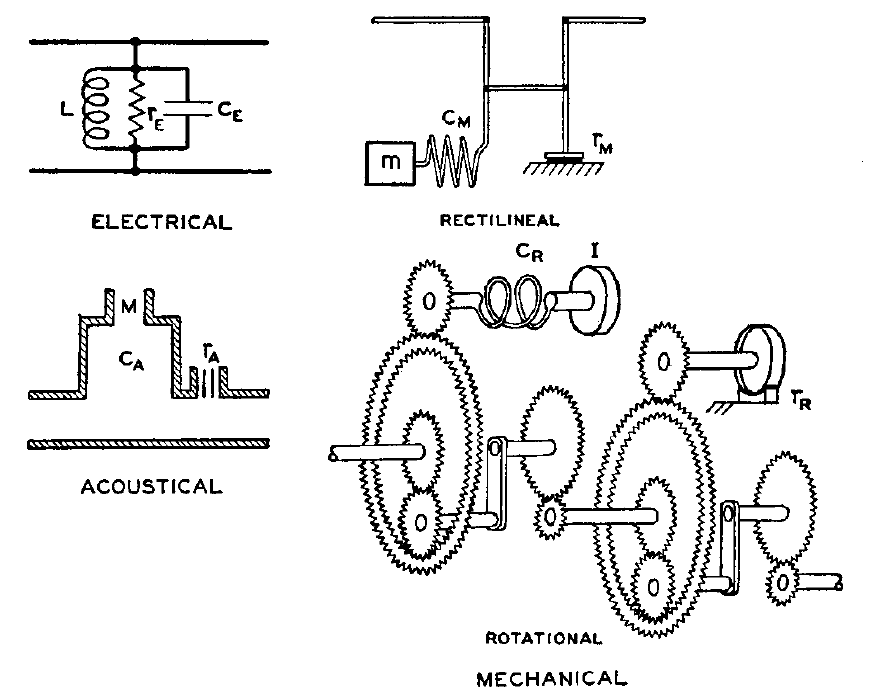By 1961, Paynter had made the analogies between these various systems mathematically precise using ‘bond graphs’:

• Henry M. Paynter, Analysis and Design of Engineering Systems, MIT Press, Cambridge, Massachusetts, 1961.

Here he shows a picture of a hydroelectric power plant, and the bond graph that abstractly describes it: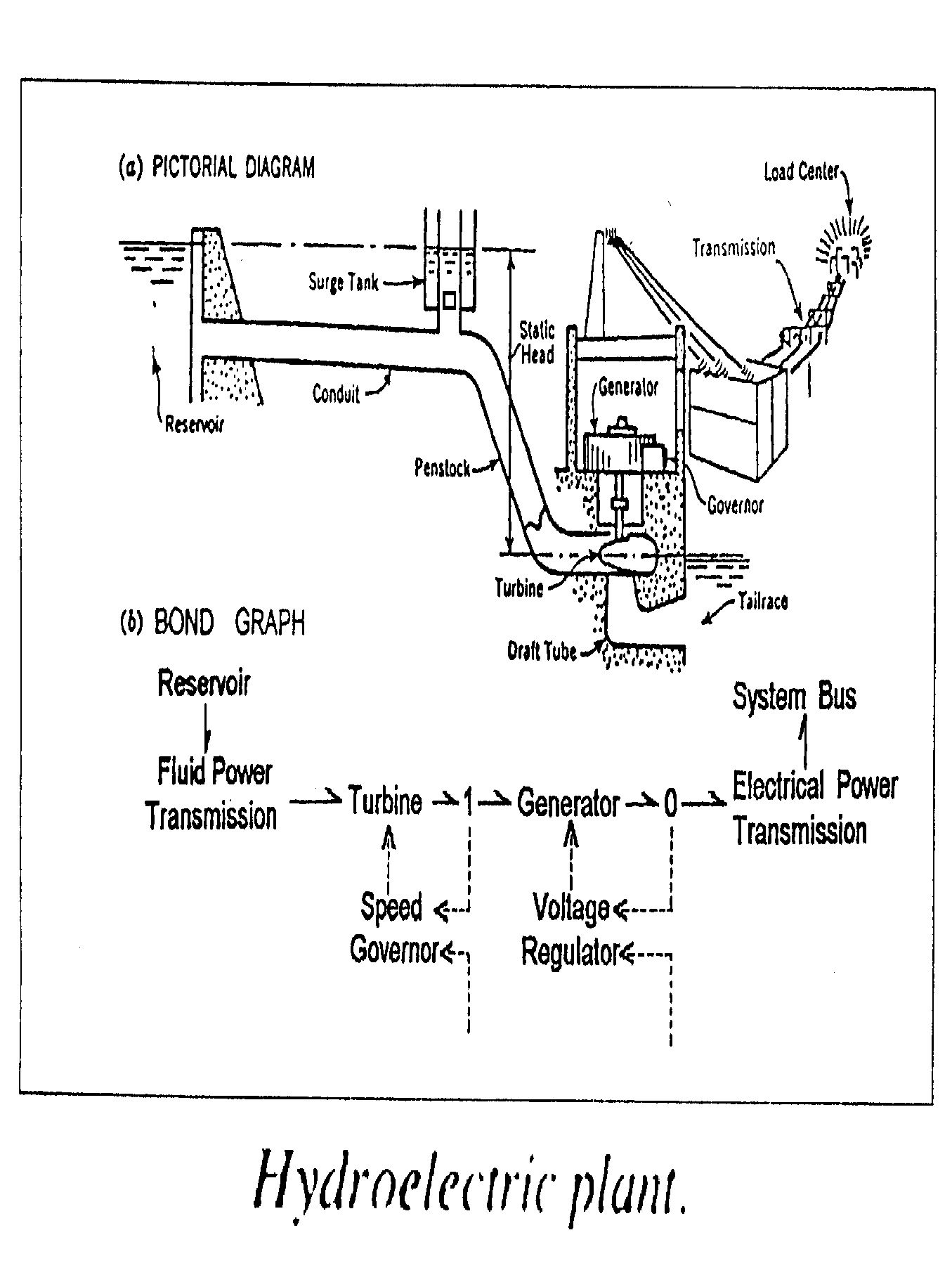By 1963, Forrester was using circuit diagrams in economics:

• Jay Wright Forrester, Industrial Dynamics, MIT Press, Cambridge, Massachusetts, 1961.

In 1984, Odum published a beautiful and influential book on their use in biology and ecology:

• Howard T. Odum, Ecological and General Systems: An Introduction to Systems Ecology, Wiley, New York, 1984.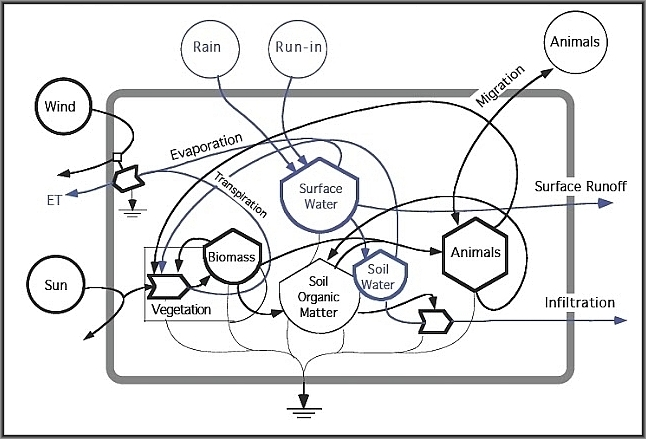We can use props to study circuit diagrams of all these kinds! The underlying mathematics is similar in each case, so we focus on just one example: electrical circuits. For other examples, take a look at this:

In our new paper, we illustrate the usefulness of props by giving a new, shorter proof of the ‘black-boxing theorem’ proved here:

A ‘black box’ is a system with inputs and outputs whose internal mechanisms are unknown or ignored. A simple example is the lock on a doorknob: one can insert a key and try to turn it; it either opens the door or not, and it fulfills this function without us needing to know its inner workings. We can treat a system as a black box through a process called ‘black-boxing’, which forgets its inner workings and records only the relation it imposes between its inputs and outputs. Systems with inputs and outputs can be seen as morphisms in a category, where composition uses the outputs of the one system as the inputs of another. We thus expect black-boxing to be a functor out of a category of this sort. A ‘black-boxing theorem’ makes this intuition precise.

In an electrical circuit, associated to each wire there is a pair of variables called the potential $\phi$ and current $I$. When we black-box such a circuit, we record only the relation it imposes between the variables on its input and output wires. Since these variables come in pairs, this is a relation between even-dimensional vector spaces. But these vector spaces turn out to be equipped with extra structure: they are symplectic vector spaces, meaning they are equipped with a nondegenerate antisymmetric bilinear form. Black-boxing gives a relation that respects this extra structure: it is a ‘Lagrangian’ relation.

Why does symplectic geometry show up when we black-box an electrical circuit? The first proof of the black-boxing theorem answered this question. A circuit made of linear resistors acts to minimize the total power dissipated in the form of heat. More generally, any circuit made of linear resistors, inductors and capacitors obeys a generalization of this ‘principle of minimum power’. Whenever a system obeys a minimum principle, it establishes a Lagrangian relation between input and output variables. This fact was first noticed in classical mechanics, where systems obey the ‘principle of least action’. Indeed, symplectic geometry has its origins in classical mechanics. But it applies more generally: for any sort of system governed by a minimum principle, black-boxing should give a functor to some category where the morphisms are Lagrangian relations.

The first step toward such a theorem for electrical circuits is to treat circuits as morphisms in a suitable category. We start with circuits made only of ideal perfectly conductive wires. These are morphisms in a prop we call $\mathrm{Circ},$ defined in Section 3 of our paper. In Section 8 we construct a black-boxing functor

$\blacksquare \colon \mathrm{Circ} \to \mathrm{LagRel}_k$

sending each such circuit to the relation it defines between its input and output potentials and currents. Here $\mathrm{LagRel}_k$ is a prop with symplectic vector spaces of the form $k^{2n}$ as objects and linear Lagrangian relations as morphisms, and $\blacksquare$ is a morphism of props. We work in a purely algebraic fashion, so $k$ here can be any field.

In Section 9 we extend black-boxing to a larger class of circuits that include linear resistors, inductors and capacitors. This gives a new proof of the black-boxing theorem that Brendan and I proved: namely, there is a morphism of props

$\blacksquare \colon \mathrm{Circ}_k \to \mathrm{LagRel}_k$

sending each such linear circuit to the Lagrangian relation it defines between its input and output potentials and currents. The ease with which we can extend the black-boxing functor is due to the fact that all our categories with circuits as morphisms are props. We can describe these props using generators and relations, so that constructing a black-boxing functor simply requires that we choose where it sends each generator, and check that the all the relations hold. In Section 10 we explain how electric circuits are related to signal-flow diagrams, used in control theory. Finally, in Section 11, we illustrate how props can be used to study nonlinear circuits.

### Outline of the results

The paper is pretty long, so here’s a more detailed outline of the results.

In Section 1 we explain a general notion of `$L$-circuit’ that was first introduced under a different name here:

An $L$-circuit is a cospan of finite sets where the apex is the set of nodes of a graph whose edges are labelled by elements of some set $L.$ In applications to electrical engineering, the elements of $L$ describe different ‘circuit elements’ such as resistors, inductors and capacitors. We discuss a symmetric monoidal category $\mathrm{Circ}_L$ whose objects are finite sets and whose morphisms are (isomorphism classes of) $L$-circuits.

In Section 2 we study $\mathrm{Circ}_L$ when $L$ is a 1-element set. We call this category simply $\mathrm{Circ}.$ In applications to engineering, a morphism in $\mathrm{Circ}$ describes circuit made solely of ideal conductive wires. We show how such a circuit can be simplified in two successive stages, described by symmetric monoidal functors:

$\mathrm{Circ} \stackrel{G}{\longrightarrow} \mathrm{FinCospan} \stackrel{H}{\longrightarrow} \mathrm{FinCorel}$

Here $\mathrm{FinCospan}$ is the category of cospans of finite sets, while $\mathrm{FinCorel}$ is the category of ‘corelations’ between finite sets. Corelations, categorically dual to relations, are already known to play an important role in network theory:

Just as a relation can be seen as a jointly monic span, a corelation can be seen as a jointly epic cospan. The functor $G$ crushes any graph down to its set of components, while $H$ reduces any cospan to a jointly epic one.

In Section 4 we turn to props. Propositions 11 and 12, proved in Appendix A.1 with the help of Steve Lack, characterize which symmetric monoidal categories are equivalent to props and which symmetric monoidal functors are isomorphic to morphisms of props. We use these to find props equivalent to $\mathrm{Circ}_L,$ $\mathrm{Circ},$ $\mathrm{FinCospan}$ and $\mathrm{FinCorel}.$ This lets us reinterpret the arrows here as morphisms of props:

$\mathrm{Circ} \stackrel{G}{\longrightarrow} \mathrm{FinCospan} \stackrel{H}{\longrightarrow} \mathrm{FinCorel}$

In Section 5 we discuss presentations of props. Proposition 19, proved in Appendix A.2 using a result of Todd Trimble, shows that the category of props is monadic over the category of signatures, $\mathrm{Set}^{\mathbb{N} \times \mathbb{N}}.$ This lets us work with props using generators and relations. We conclude by recalling a presentation of $\mathrm{FinCospan}$ due to Lack and a presentation of $\mathrm{FinCorel}$ due to Coya and Fong:

In Section 6 we introduce the prop $\mathrm{FinRel}_k.$ This prop is equivalent to the symmetric monoidal category with finite-dimensional vector spaces over the field $k$ as objects and linear relations as morphisms, with direct sum as its tensor product. A presentation of this prop was given in these papers:

• Filippo Bonchi, Pawel Sobociénski and Fabio Zanasi, Interacting Hopf algebras, Journal of Pure and Applied Algebra 221 (2017), 144–184.

• John Baez and Jason Erbele, Categories in control, Theory and Applications of Categories 30 (2015), 836–881. (Blog article here.)

In Section 7 we state the main result in the paper by Rosebrugh, Sabadini and Walters. This gives a presentation of $\mathrm{Circ}_L.$ Equivalently, it says that $\mathrm{Circ}_L$ is the coproduct, in the category of props, of $\mathrm{FinCospan}$ and the free prop on a set of unary operations, one for each element of $L.$ This result makes it easy to construct morphisms from $\mathrm{Circ}_L$ to other props.

In Section 8 we introduce the prop $\mathrm{LagRel}_k$ where morphisms are Lagrangian linear relations between symplectic vector spaces, and we construct the black-boxing functor $\blacksquare \colon \mathrm{Circ} \to \mathrm{LagRel}_k.$ Mathematically, this functor is the composite

$\mathrm{Circ} \stackrel{G}{\longrightarrow} \mathrm{FinCospan} \stackrel{H}{\longrightarrow} \mathrm{FinCorel} \stackrel{K}{\longrightarrow} \mathrm{LagRel}_k$

where $K$ is a symmetric monoidal functor defined by its action on the generators of $\mathrm{FinCorel}.$ In applications to electrical engineering, the black-boxing functor maps any circuit of ideal conductive wires to its ‘behavior’: that is, to the relation that it imposes on the potentials and currents at its inputs and outputs.

In Section 9 we extend the black-boxing functor to circuits that include resistors, inductors, capacitors and certain other linear circuit elements. The most elegant prop having such circuits as morphisms is $\mathrm{Circ}_k,$ meaning $\mathrm{Circ}_L$ with the label set $L$ taken to be a field $k.$ We characterize the black-boxing functor

$\blacksquare \colon \mathrm{Circ}_k \to \mathrm{LagRel}_k$

in Theorem 41.

In Section 10 we expand the scope of inquiry to include ‘signal-flow diagrams’, which are important in control theory. We explain how signal-flow diagrams serve as a syntax for linear relations. Concretely, this means that signal-flow diagrams are morphisms in a free prop $\mathrm{SigFlow}_k$ with the same generators as $\mathrm{FinRel}_k,$ but no relations. There is thus a morphism of props

$\square \colon \mathrm{SigFlow}_k \to \mathrm{FinRel}_k$

mapping any signal-flow diagrams to the linear relation that it denotes. It is natural to wonder how this is related to the black-boxing functor

$\blacksquare \colon \mathrm{Circ}_k \to \mathrm{LagRel}_k$

The answer involves the free prop $\widetilde{\mathrm{Circ}}_k$ which arises when we take the simplest presentation of $\mathrm{Circ}_k$ and omit the relations. This comes with a map

$P \colon \widetilde{\mathrm{Circ}}_k \to \mathrm{Circ}_k$

which reinstates those relations, and in Theorem 44 we show there is a map of props $T \colon \widetilde{\mathrm{Circ}}_k \to \mathrm{SigFlow}_k$ making this diagram commute: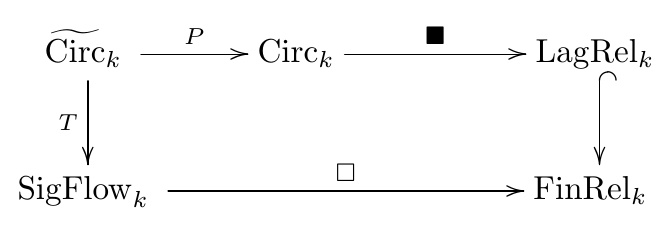Putting everything together, we get this grand commuting diagram relating circuit diagrams, linear circuits, signal flow diagrams, cospans, corelations, Lagrangian relations, and linear relations: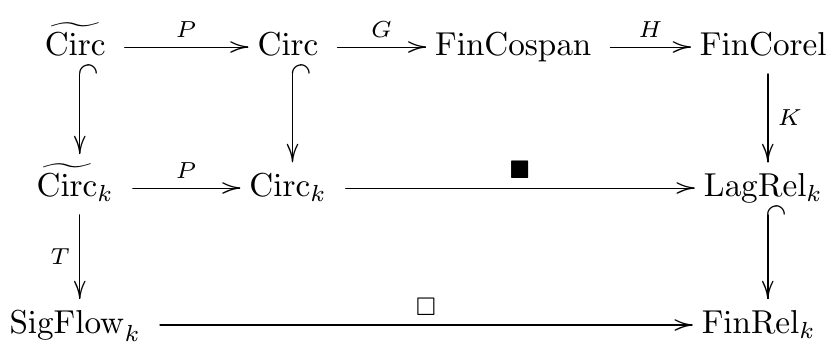Finally, in Section 11 we illustrate how props can also be used to study nonlinear circuits. Namely, we show how to include voltage and current sources. Black-boxing these gives Lagrangian affine relations between symplectic vector spaces! Eventually we’ll get around to studying more general nonlinear circuits… but not today.

Posted at April 27, 2018 4:59 PM UTC

TrackBack URL for this Entry:   https://golem.ph.utexas.edu/cgi-bin/MT-3.0/dxy-tb.fcgi/3033

### Re: Props in Network Theory

There’s something wrong with the URL for the Joyal and Street paper.

Posted by: carl feynman on April 29, 2018 5:58 PM | Permalink | Reply to this

### Re: Props in Network Theory

Posted by: Jesse C. McKeown on April 30, 2018 1:22 AM | Permalink | Reply to this

### Re: Props in Network Theory

Posted by: John Baez on April 30, 2018 6:28 AM | Permalink | Reply to this

### Re: Props in Network Theory

Hopf (and Frobenius) algebras feature in the presentation of props. These structures also underlie the work of Coecke, Kartsaklis and their colleagues on distributional and compositional models of language. If I am reading the empirical chapter of Karsaklis’s PhD properly, prior disambiguation of sentences does not seem to effect much improvement when applied to machine learning algorithms. This suggests to me that convolution neural networks have the capacity to capture the deep mathematical structures expressed by these algebras and their interactions. Yet there is nothing in the literature on this capability (other than some recent discussions of robustness properties based on regularization theory). Perhaps regularization theory is in need of a major shake-up along similar lines to those you are investigating for circuit models! Any thoughts on this?

Posted by: James Juniper on May 5, 2018 3:58 AM | Permalink | Reply to this

### Re: Props in Network Theory

I don’t know much about compositional or distributional models of language, though I’ve recently learned a bit from the Applied Category Theory 2018 school and workshop—and these blog posts by students at the school:

What I know is that anytime you’re dealing with relations (in the mathematical sense), bialgebras are lurking in the background, since the prop $FinRel$, where a morphism $f : m \to n$ is a relation between an $m$-element set and an $n$-element set, is the prop for extraspecial bicommutative bialgebras. And anytime you’re dealing with corelations, Frobenius algebras are lurking in the background, since the prop $FinCorel$, where a morphism is a corelation between an $m$-element set and an $n$-element set, is the prop for extraspecial commutative Frobenius monoids.

So, bialgebras and Frobenius algebras are very fundamental structures that we should expect to see all over the place.

(See the stuff around example 17 in our paper for more on this, including explanations of all the jargon.)

Posted by: John Baez on May 5, 2018 10:21 AM | Permalink | Reply to this

### Re: Props in Network Theory

It is mentioned above (or at least alluded to) that FinCospan is the prop for special commutative Frobenius algebras. (See the paper for details; the result is due to Lack and Rosebrugh-Sabatini-Walters.) ‘Special’ means that comultiplication followed by multiplication is the identity. Topologically, this is sort of a sad equation, because it is a loop that is suppressed :-(

To see the loop, let the comultiplication be you left hand with thumb and forefinger as outputs, and let the multiplication be your right hand with thumb and forefinger as inputs. Now compose, and you are staring into the loop. In terms of cospans, it is

{elbow} –> {wrist} <– {thumb-tip,forefingertip}

composed with

{thumb-tip,forefingertip} –> {wrist} <– {elbow}

The composition is by pushout, it’s the pushout of the span

{wrist} <– {thumb-tip,forefingertip} –> {wrist}

in the category of sets, yielding a singleton.

What about general (nonspecial) commutative Frobenius algebras?

The ‘problem’ is with the pushout. Although the category of sets is cocomplete, some of its colimits are not optimal from a homotopical viewpoint. This pushout is one example of a bad colimit. (See for example John’s recent blog post here.)

If we take a homotopy pushout instead, things look different: recall that the homotopy pushout of a span A <– M –> B is given by ‘sewing in a path’ in A+B for each point in M. If you still have your hands in the position of the composite, you can see these paths: they are formed by your fingers: one path joining your wrists via the thumbs and one path joining your wrists via the forefingers. Together they form indeed a loop!

Now, of course, it makes no sense to talk about paths in the category of sets, so we need a more homotopical context. The minimal context for the present purposes is that of 1-dimensional CW-complexes, i.e. topological graphs. So to make sense of our finite cospans and homotopy pushouts, we need cospans where the apex is a 1-dimensional CW-complex, but we can keep the endpoints of the cospans as finite sets as before. To see that this works, note that the (homotopy) pushouts taken when composing such cospans are always over a finite set, so that the effect is to sew in a finite number of paths between 1-dimensional CW-complexes. This yields a 1-dimensional CW-complex again.

Note that cospans whose apexes are graphs appear already in Rosebrugh-Sabatini-Walters, but there the graphs are combinatorial graphs. Here they are topological graphs, and they are considered up to homotopy only. Indeed, to make this thing into an ordinary prop, one must consider homotopy classes of cospans.

Now you can guess the result:

Theorem: this prop is the prop for commutative Frobenius algebras.

A proof (sketch) can be found in this note with David Spivak: Homotopy composition of cospans. The paper is only 4 pages long :-)

So what’s next?

Somehow, the homotopy result ought to be the real thing, from which the special result is obtained by the brutal act of taking $\pi_0$.

Unfortunately, I don’t know of any situations in network theory where the homotopy refinement seems useful :-( To the contrary, if you look at electrical networks, the special Frobenius structure encodes the copper wires. And what the electrical current and potential care about is whether there is a copper connection or not, not whether there is a copper loop they could have fun with. And if you go to categorical quantum mechanics, where famously classical structure (say classical communication channels) are encoded as special commutative Frobenius algebras, the experts will tell you that it is essential that the Frobenius algebras are special. One of the basic theorems in the theory is the spider theorem which says that for a connected graph with $m$ inputs and $n$ outputs, only the $m$ inputs and $n$ outputs matters, not the loop number – and such a thing is called a spider, in analogy with the $(m+n)$-legged spiders found in nature.

So this is a call for help: do you know of some situation in network theory where you would like to care about loops?

(Speaking of fun, perhaps when you were a child you lived in a simply-connected home. But sometimes you would visit someone in a non-simply-connected flat, and you would enjoy very much to run around and around, from the kitchen to the dining room to the corridor and from there into to the kitchen, and then into the dining room, and so on. Fantastic fun!)

Posted by: Joachim Kock on May 5, 2018 8:16 PM | Permalink | Reply to this

### Re: Props in Network Theory

Joachim wrote:

It is mentioned above (or at least alluded to) that FinCospan is the prop for special commutative Frobenius algebras.

Yes, Example 17 of our paper discusses these nice known results:

• FinCospan is the prop for special bicommutative Frobenius algebras.
• FinCorel is the prop for extraspecial bicommutative Frobenius algebras.
• FinSpan is the prop for extra bicommutative bialgebras.
• FinRel is the prop for extraspecial bicommutative bialgebras.

(Any commutative Frobenius algebra is automatically bicommutative, and every bialgebra automatically obeys the ‘extra’ identity, but this way of stating the results makes the pattern clear. I should also add, for nonexperts, that the word ‘algebra’ can be replaced by the word ‘monoid’ without changing the meaning: we’re not doing linear algebra here.)

Having gone this far it’s fascinating to look at generalizations, like the prop for commutative Frobenius algebras—our friend 2Cob, which you’re now describing in a new homotopical way. Some other fun ones are the prop for bialgebras and the prop for bicommutative bialgebras, which are discussed here.

Unfortunately, I don’t know of any situations in network theory where the homotopy refinement seems useful :-( To the contrary, if you look at electrical networks, the special Frobenius structure encodes the copper wires. And what the electrical current and potential care about is whether there is a copper connection or not, not whether there is a copper loop they could have fun with.

The loops in copper wire become important when we consider alternating currents: then an alternating current in one loop creates an oscillating magnetic field which can induce an alternating current in another loop linked to the first one! This is roughly how ‘inductors’ work, which is why we see gizmos like this, some with very large linking numbers:All this suggests it might be nice to look at the braided monoidal category of topological graphs embedded in $\mathbb{R}^3$, which should be something like the free braided monoidal category on a commutative Frobenius algebra. This is not a prop but rather a ‘prob’. Do you have ways of studying these?

(Speaking of fun, perhaps when you were a child you lived in a simply-connected home. But sometimes you would visit someone in a non-simply-connected flat, and you would enjoy very much to run around and around, from the kitchen to the dining room to the corridor and from there into to the kitchen, and then into the dining room, and so on. Fantastic fun!)

Yes it’s true! The practical-minded grownups were content to know $\pi_0$ of the house, it seems, but kids enjoy $\pi_1$. I recently saw a room with nonzero $\pi_2$ and was utterly amazed and delighted. Click the picture for details:This room also had nontrivial $\pi_3$, but I didn’t notice that until just now.

Posted by: John Baez on May 7, 2018 6:07 AM | Permalink | Reply to this

Post a New Comment